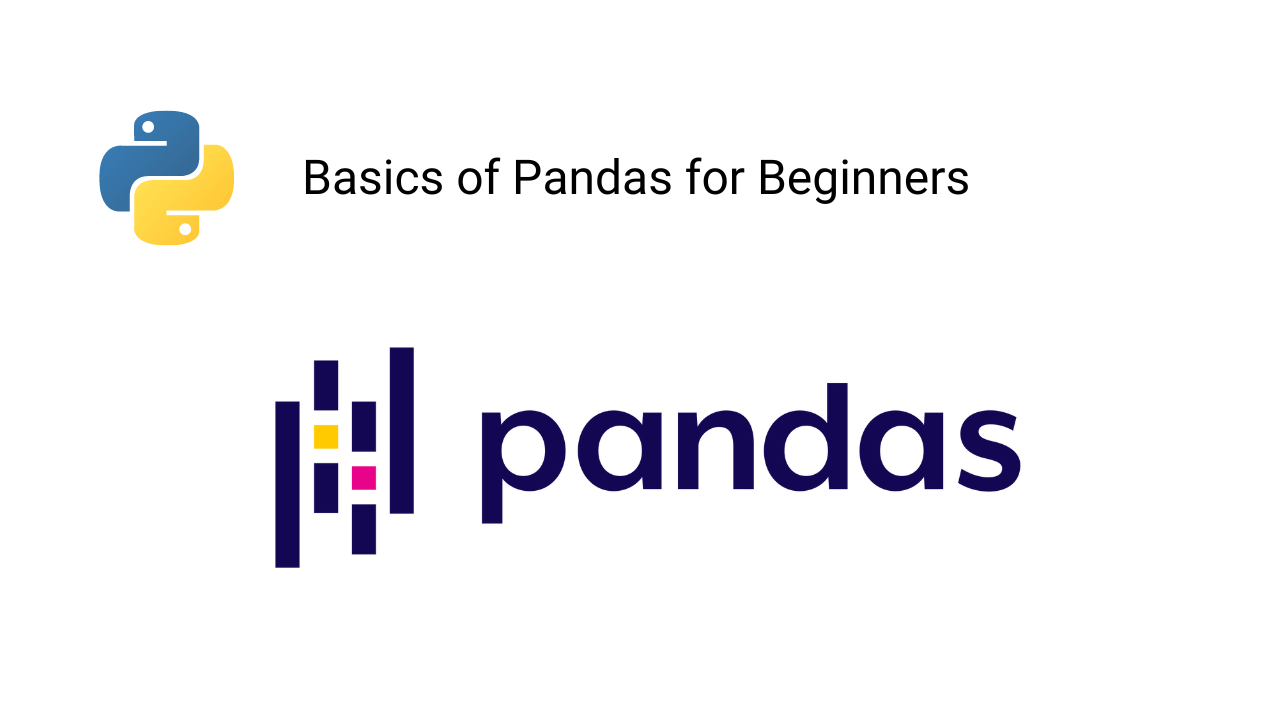# pythonIntroduction to Pandas Library in Python

Pandas is a well-known Python library widely utilized by data scientists and analysts for managing, processing, and analyzing data. This library offers a vast array of efficient tools and functions to streamline data manipulation and analysis. In this blog, we will guide you through the installation process and provide an overview of the most frequently used basic Pandas functions in machine learning projects.Introduction to NumPy in Python

Numpy is widely recognized as one of the most essential Python libraries for building machine learning and data science applications. Its ability to perform parallel computing and execute certain functions using C programming language makes it lightning fast. Numpy array is a data structure used to store homogenous datatype and it is different from python lists and python arrays. In this article, we will cover the basics of the Numpy library, including installation and the most commonly used functions for executing mathematical operations.Data Types and Variables in Python Programming

Python is the most preferred language for developing machine learning and data science applications. It has a large community support that can help debug the errors and resolve all the roadblocks appearing while developing any solution. In this blog, we have discussed various data types like integers, floats, boolean and strings along with their usage in Machine Learning and Data Science.Conditions and Branching in Python

We sometimes need to execute specific instructions only when some conditions are true. If not, then we will perform a different set of instructions. In this blog, we have discussed: 1) Various comparison operations in Python. 2) What are conditions in python? 3) What is branching? 3) How do we use logical operations to combine the two conditions? etc.Loop in Python Programming

Loops are the set of instructions that needs to be executed repeatedly until a defined condition is satisfied. In this blog, we have discussed the range function in python working of a loop and the most popular loops: for and while loop in python. The use of continue and break statements in loop increase their usability in Python and building ML Applications.Functions in Python Programming

Functions are a set of instructions grouped in a block and get executed only when it is called inside our program. In python programming, functions follow specific syntaxes to ensure their validity. In this blog, we have discussed: What are functions in python? How to create and call functions? Various types of function arguments, The anonymous function and most used python in-built functions in ML and Data Science projects.Classes and Objects in Python

In Python, everything is an object which hold different properties and methods. Class is a blueprint that creates these objects. In this blog, we will discuss more about the classes and objects in Python.Introduction to Seaborn in Python

Seaborn is an open-source library built over Matplotlib and makes plots more appealing and understandable. It works excellently with data frames and pandas libraries. In this blog, we have discussed: 1) Advantages of Seaborn over Matplotlib library, 2) Installation process of Seaborn in Python 3) Various Data Plots using the Seaborn library.Matplotlib Library in Python

Matplotlib is one of Python's most effective visualization libraries for data visualization. It is an open-source library built over NumPy arrays. In this blog, we have discussed: 1) What is Matplotlib 2) Installation of Matplotlib using PIP 3) What is Pyplot in Matplotlib 4) The subplot in Matplotlib's pyplot module 5) Various plots using Matplotlib.Sets and Dictionaries in Pyhton Programming

In python, sets and dictionaries are unordered data structures frequently used in machine learning applications. In this blog, we have explained these concepts: 1) What is set in python? 2) Important operations on sets, 3) Conversion of lists into sets 4) What is dictionary in python? 5) Operations on dictionaries? 6) Comparison of sets and dictionaries.Tuples and Lists in Python Programming

Tuples and lists are two most popular python data structures used in Machine Learning and Data Science applications. They are also called compound data types because they can store a mixture of primitive data types like strings, ints, and floats. Tuples are static and immutable while lists are dynamic and mutable, but tuples are memory efficient as compared to lists. We will explore these data structures in detail in this blog.Python Date and Time

As data scientists, we should know how to handle the date-time data and the standard set of date-time operations we can apply to transform the raw data. Fortunately, we have date-time manipulation libraries specifically for this purpose. In this blog, we will talk about all basic date-time manipulations, explorations, transformations, and applications.